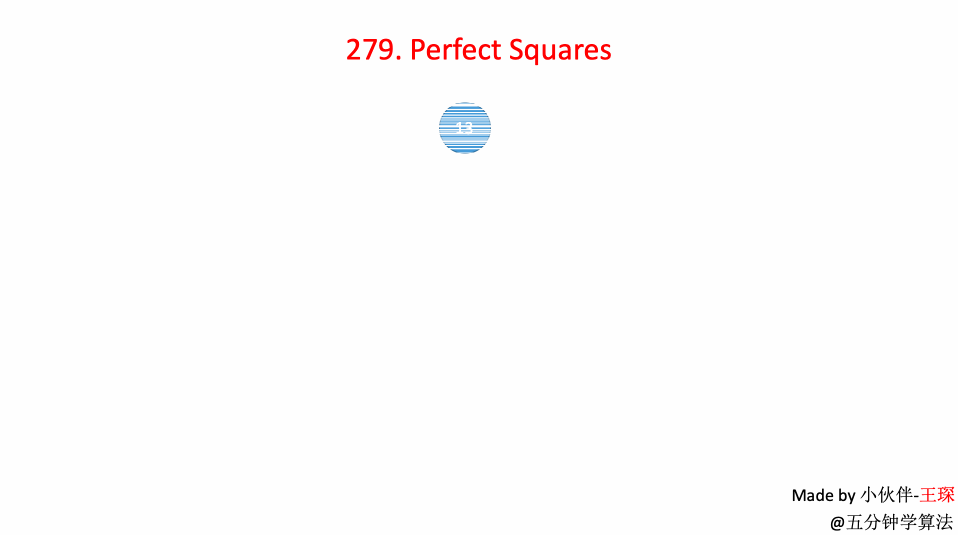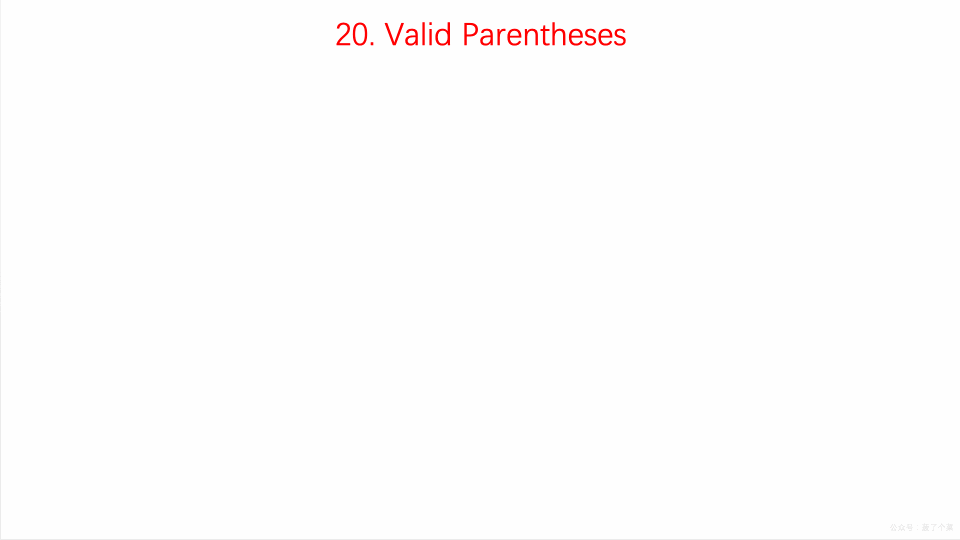1、从图中某个顶点 V出发，并访问此顶点；

2、从 V出发，访问 V的各个 未被访问 的邻接点 W1， W2，…， W；然后依次从 W1， W2，…， Wk 出发访问各自未被访问的邻接点；

3、重复步骤 2 ，直到全部顶点都被访问为止。

## 1 二叉树的层次遍历

### 代码实现

public List<List<Integer>> levelOrder(TreeNode root) {    if(root == null)        return new ArrayList<>();    List<List<Integer>> res = new ArrayList<>();    Queue<TreeNode> queue = new LinkedList<TreeNode>();    queue.add(root);    while(!queue.isEmpty()){        int count = queue.size();        List<Integer> list = new ArrayList<Integer>();        while(count > 0){            TreeNode node = queue.poll();            list.add(node.val);            if(node.left != null)                queue.add(node.left);            if(node.right != null)                queue.add(node.right);            count--;        }        res.add(list);    }    return res;}

## 2 完全平方数

### 题目解析

public int numSquares(int n) {        while (n % 4 == 0){            n /= 4;        }        if ( n % 8 == 7){            return 4;        }        int a = 0;        while ( (a * a) <= n){            int b = (int)Math.pow((n - a * a),0.5);             if(a * a + b * b == n) {            //如果可以 在这里返回            if(a != 0 && b != 0) {                return 2;            } else{                return 1;            }        }        a++;     }        return 3;}

### 动画演示### 代码实现

import java.util.LinkedList;import javafx.util.Pair;class Solution {    public int numSquares(int n) {         if(n == 0)            return 0;        LinkedList<Pair<Integer, Integer>> queue = new LinkedList<Pair<Integer, Integer>>();        queue.addLast(new Pair<Integer, Integer>(n, 0));        boolean[] visited = new boolean[n+1];        visited[n] = true;        while(!queue.isEmpty()){            Pair<Integer, Integer> front = queue.removeFirst();            int num = front.getKey();            int step = front.getValue();            if(num == 0)                return step;            for(int i = 1 ; num - i*i >= 0 ; i ++){                int a = num - i*i;                if(!visited[a]){                    if(a == 0) return step + 1;                    queue.addLast(new Pair(num - i * i, step + 1));                    visited[num - i * i] = true;                }            }        }        return 0;    }}

## 3 员工的重要性

### 题目描述

输入: [[1, 5, [2, 3]], [2, 3, []], [3, 3, []]], 1输出: 11解释:员工 1 自身的重要度是 5，他有两个直系下属 2 和 3 ，而且 2 和 3 的重要度均为 3 。因此员工 1 的总重要度是 5 + 3 + 3 = 11。

### 代码实现

public int getImportance(List<Employee> employees, int id) {        Employee emp = null;        //重要度        int sum = 0;           //存储员工信息        HashMap<Integer,Employee> map=new HashMap<Integer,Employee>();  /        for(Employee e:employees) {            map.put(e.id, e);        }        //使用广度优先遍历员工        ArrayDeque<Employee> queue=new ArrayDeque<Employee>();        queue.addLast(map.get(id));        while(!queue.isEmpty()) {            emp=queue.removeFirst();            sum+=emp.importance;            for(int i:emp.subordinates) {                queue.addLast(map.get(i));            }        }        return sum;    }

## 4 删除无效的括号

### 题目描述

输入: "()())()"输出: ["()()()", "(())()"]

输入: "(a)())()"输出: ["(a)()()", "(a())()"]

输入: ")("输出: [""]

### 动画演示public class Solution {    public List<String> removeInvalidParentheses(String s) {        queue.offer(s);        vis.add(s);        boolean flag=false;        List<String> ans=new ArrayList<String>();        while (!queue.isEmpty()){            String cur=queue.poll();            if(flag){                check(cur,ans);            }else{                flag=checkLeft(cur,ans)||checkRight(cur,ans);            }        }        if(ans.size()==0){            ans.add("");        }        return new ArrayList<String>(ans);    }    Set<String> vis=new HashSet<String>();    Queue<String> queue=new LinkedList<String>();    public void check(String s,List<String> ans){  //查看是否正确        Stack<Character> st=new Stack<Character>();        for(char c:s.toCharArray()){            if(c=='('){                st.push(c);            }            if(c==')'){                if(st.isEmpty()){                    return;                }                st.pop();            }        }        if(st.isEmpty()){            ans.add(s);        }    }    public boolean checkLeft(String s,List<String> ans){ //检查左边        //delete right        Stack<Character> st=new Stack<Character>();        for(int i=0;i<s.length();++i){            if(s.charAt(i)=='('){                st.push(s.charAt(i));            }else if(s.charAt(i)==')'){                if(st.isEmpty()){                    for(int j=i;j>=0;--j){ //删除不符合的')'  多种情况                        if(s.charAt(j)==')'){                            String s1=s.substring(0,j)+s.substring(j+1);                            if(!vis.contains(s1)){                                vis.add(s1);                                queue.offer(s1);                            }                        }                    }                    return false;                }else{                    st.pop();                }            }        }        if(st.isEmpty()){            ans.add(s);            return true;        }        return false;    }    public boolean checkRight(String s,List<String> ans){ //检查右边        //delete right        Stack<Character> st=new Stack<Character>();        st.clear();        for(int i=s.length()-1;i>=0;--i){            if(s.charAt(i)==')'){                st.push(s.charAt(i));            }else if(s.charAt(i)=='('){                if(st.isEmpty()){                    for(int j=i;j<s.length();++j){                        if(s.charAt(j)=='('){  //删除不符合的'(' 多种情况                            String s1=s.substring(0,j)+s.substring(j+1);                            if(!vis.contains(s1)){                                vis.add(s1);                                queue.add(s1);                            }                        }                    }                    return false;                }else{                    st.pop();                }            }        }        if(st.isEmpty()){            ans.add(s);            return true;        }        return false;    }}